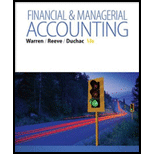# Profitability ratios Ralph Lauren Corporation sells apparel through company-owned retail stores. Recent financial information for Ralph Lauren follows (in thousands): Fiscal Year 3 Final Year 2 Net income $567,600$479,500 Interest expense 18,300 22,200 Fiscal Year 3 Fiscal Year 2 Fiscal Year 1 Total assets (at end of fiscal year) $4,981,100$4,648,900 $4,356,500 Total stockholders' equity {at end of fiscal year) 3,304,700 3,116,600 2,735,100 Assume the apparel industry average return on total assets is 8.0%, and the average rate earned on stockholders' equity is 10.0% for the year ended April 2, Year 3. A. Determine the return on total assets for Ralph Lauren for fiscal Years 2 and 3. (Round percentages to one decimal place.) B. Determine the return on stockholders’ equity for Ralph Lauren for fiscal Years 2 and 3. (Round percentages to one decimal place.) C. Evaluate the two-year trend for the profitability ratios determined in (A) and (B). D. Evaluate Ralph Lauren s profit performance relative to the industry.BuyFindarrow_forward ### Financial & Managerial Accounting 13th Edition Carl Warren + 2 others Publisher: Cengage Learning ISBN: 9781285866307 #### Solutions Chapter SectionBuyFindarrow_forward ### Financial & Managerial Accounting 13th Edition Carl Warren + 2 others Publisher: Cengage Learning ISBN: 9781285866307 Chapter 15, Problem 15.18EX Textbook Problem 40 views ## Profitability ratiosRalph Lauren Corporation sells apparel through company-owned retail stores. Recent financial information for Ralph Lauren follows (in thousands): Fiscal Year 3 Final Year 2 Net income$567,600 $479,500 Interest expense 18,300 22,200 Fiscal Year 3 Fiscal Year 2 Fiscal Year 1 Total assets (at end of fiscal year)$4,981,100 $4,648,900$4,356,500 Total stockholders' equity {at end of fiscal year) 3,304,700 3,116,600 2,735,100 Assume the apparel industry average return on total assets is 8.0%, and the average rate earned on stockholders' equity is 10.0% for the year ended April 2, Year 3. A. Determine the return on total assets for Ralph Lauren for fiscal Years 2 and 3. (Round percentages to one decimal place.) B. Determine the return on stockholders’ equity for Ralph Lauren for fiscal Years 2 and 3. (Round percentages to one decimal place.) C. Evaluate the two-year trend for the profitability ratios determined in (A) and (B). D. Evaluate Ralph Lauren s profit performance relative to the industry.

a)

To determine

Financial Ratios: Financial ratios are the metrics used to evaluate the liquidity, capabilities, profitability, and overall performance of a company.

To determine: Return on total assets for RL for fiscal years 2 and 3

Given info: Selected items from the financial statements for fiscal years 1, 2, and 3.

### Explanation of Solution

• Determine return on total assets.

Fiscal year 3

Rate of return on assets=Netincome + Interest expenseAverage total assets=$567,600+$18,300$4,815,000=12.2% Fiscal year 2 Rate of return on assets=Netincome + Interest expenseAverage total assets=$479,500+$22,200$4,502,700=11.1%

Return on assets determines the particular company’s overall earning power. It is determined by dividing sum of net income and interest expense and average total assets

b)

To determine

To Determine: return on stockholders’ equity.

c)

To determine

To evaluate: Two-year trend for the profitability ratios.

d)

To determine

To evaluate: RL’s profit performance relative to the industry

### Still sussing out bartleby?

Check out a sample textbook solution.

See a sample solution

#### The Solution to Your Study Problems

Bartleby provides explanations to thousands of textbook problems written by our experts, many with advanced degrees!

Get Started

Find more solutions based on key concepts
In what ways is economics a science?

Brief Principles of Macroeconomics (MindTap Course List)

Discuss target market strategies

MKTG 12:STUDENT ED.-TEXT

ALTERNATIVE DIVIDEND POLICIES Rubenstein Bros. Clothing is expecting to pay an annual dividend per share of 0.7...

Fundamentals of Financial Management, Concise Edition (with Thomson ONE - Business School Edition, 1 term (6 months) Printed Access Card) (MindTap Course List)